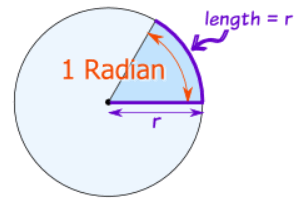# Graphing Waves

Standard

In these two videos we learn how to graph waves using trigonometric functions.

# Graphs of Sine and Cosine

Standard

In these videos we learn about the graphs of sin(x) and cos(x).

# Reciprocal Trig Ratios

Standard

In this video we learn three new trig ratios called the reciprocal ratios. (secant,cosecant,and cotangent)

# Unit Circle Part 2

Standard

More about why the unit circle is useful.

# The Unit Circle

Standard

In this video I introduce the unit circle.

Standard

A radian is a unit of measuring angles just like a degree is. A radian is a distance on the circle’s circumference which is equal to 1 radius.This means there are$2\pi$ radians in one circle or that$2\pi$ radians equals 360 degrees.

If$2\pi$ radians equals 360 degrees that means that 1 degree equals$2\pi$ divided by 360 radians or$\pi$ divided by 180 radians.

1 radian =$\frac{180}{\pi}$ degrees

1 degree =$\frac{\pi}{180}$ radiansThis is a degrees and radians chart. If 1 degree =$\frac{\pi}{180}$radians then 30 degrees equals 30 times that or$\frac{\pi}{6}$ radians. The same thing can be done to find the radian measure for other angles.

# Solving Using Trigonometry Part 2

Standard

More about solving for the side of a triangle using trigonometry.